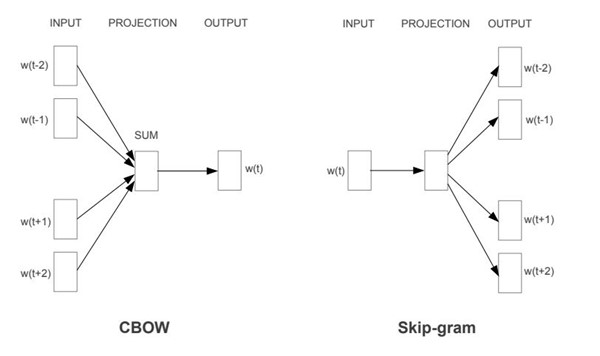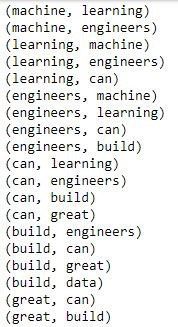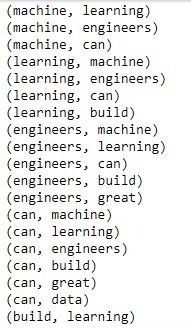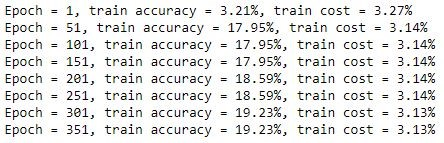Tensorflow Word2Vec Tutorial From Scratch

Word2Vec is a widely used model for converting words into a numerical representation that machine learning models can utilize known as word embeddings. In this tensorflow tutorial you will learn how to implement Word2Vec in TensorFlow using the Skip-Gram learning model.

If you are just getting started with Word2Vec, you can read the following articles to help you catch up:

Word2Vec Skip-Gram Learning Refresher

If you recall, the Skip-Gram Model can be thought as learning word embeddings by training a model to predict context given a word.

Given a an input word w(t), our tensorflow implemented neural network will be trained to predict context words. Context referring to words that co-occur with our target word. Window Size defines how many words before and after the target word to be used as context.Once our 3 layer neural network is trained, we will only care about our hidden layer which represents our word embeddings.

Let’s get started with our basic implementation of Skip-Gram, 3 layer neural network with tensorflow.

Pre-Requisites

First task is to prepare our data. We will be training the word2vec model with the following 4 sentences taken from twitter to keep things simple.

SENTENCES = [
"machine learning engineers can build great data models",
"the more data you have the better your model",
"these predictions sound right, but it is all about your data",
"your data can provide great value"
]

These are easy to use sentences, words separated by space with no special characters that would require advanced cleaning.

We will be using my Vocabulary class which implements many of the data preprocessing needed. You can also find this class in my github repository:

from collections import Counter
import json
import numpy as np
class Vocabulary:

def __init__(self, vocabulary, wordFrequencyFilePath):
self.vocabulary = vocabulary
self.BAG_OF_WORDS_FILE_FULL_PATH = wordFrequencyFilePath
self.input_word_index = {}
self.reverse_input_word_index = {}

self.input_word_index["START"] = 1
self.input_word_index["UNKOWN"] = -1
self.MaxSentenceLength = None

def PrepareVocabulary(self,reviews):
self._prepare_Bag_of_Words_File(reviews)
self._create_Vocab_Indexes()

self.MaxSentenceLength = max([len(txt.split(" ")) for txt in reviews])

def Get_Top_Words(self, number_words = None):
if number_words == None:
number_words = self.vocabulary

counter = Counter(chars)
most_popular_words = {key for key, _value in counter.most_common(number_words)}
return most_popular_words

def _prepare_Bag_of_Words_File(self,reviews):
counter = Counter()
for s in reviews:
counter.update(s.split(" "))

with open(self.BAG_OF_WORDS_FILE_FULL_PATH, 'w') as output_file:
output_file.write(json.dumps(counter))

def _create_Vocab_Indexes(self):
INPUT_WORDS = self.Get_Top_Words(self.vocabulary)

#word to int
for i, word in enumerate(INPUT_WORDS):
self.input_word_index[word] = i

#int to word
for word, i in self.input_word_index.items():
self.reverse_input_word_index[i] = word

def _word_to_One_Hot_Vector(self, word):
vector = np.zeros(self.vocabulary)
vector[vocab.input_word_index[word]] = 1
return vector

def TransformSentencesToId(self, sentences):
vectors = []
for r in sentences:
words = r.split(" ")
vector = np.zeros(len(words))

for t, word in enumerate(words):
if word in self.input_word_index:
vector[t] = self.input_word_index[word]
else:
pass
#vector[t] = 2 #unk
vectors.append(vector)

return vectors

def ReverseTransformSentencesToId(self, sentences):
vectors = []
for r in sentences:
words = r.split(" ")
vector = np.zeros(len(words))

for t, word in enumerate(words):
if word in self.input_word_index:
vector[t] = self.input_word_index[word]
else:
pass
#vector[t] = 2 #unk
vectors.append(vector)

return vectors

Bag of Words

First step is to create our bag of words and 2 important dictionaries we will need. A dictionary for going from a word to an integer and its reverse, a dictionary for going from an int to a word. Our vocabulary class will contain and populate these 2 dictionaries. They are called input_word_index and reverse_input_word_index.

Initialize the vocabulary class and call the PrepareVocabulary method to generate these. Our vocabulary will consist of 26 words (all of the words in the input sentences).

VOCABULARY_SIZE = 26
vocab = Vocabulary(VOCABULARY_SIZE,"bag_of_words.vocab")
vocab.PrepareVocabulary(SENTENCES)
print("Vocabulary of {0} words".format(len(vocab.Get_Top_Words())))

The last method will print the total number of words in our bag of words.

To get the top 5 words in our bag of words, call the Get_Top_Words method as below.

vocab.Get_Top_Words(5)

You will now see the top 5 words in our bag of words which are: {'can', 'data', 'great', 'the', 'your'}.

To convert a word in our bag of words to a integer or vice versa we use our input_word_index and reverse_input_word_index dictionary as below.

print(vocab.input_word_index["great"])
print(vocab.reverse_input_word_index)

Skip-Gram Context

With our bag of words model created, we will now define our training X and Y variables. In Skip-Gram learning model, we input a word and try to predict its context. Therefore, our X and Y variable will be a pair of target word and context word. Each target word will repeat according to other words in its context. The variable that defines how many words before and after a target word to use as input is Window Size. Use the Get_SkipGram_Target_Words function to generate these pairs.

As a sample, generate the word pairs using a Window Size of 2.

Skip_Gram_Target_Words = vocab.Get_SkipGram_Target_Words(SENTENCES, WINDOW_SIZE=2)

for target, context in Skip_Gram_Target_Words:
print("({0}, {1})".format(target,context))To increase the number of words before an after a target word is used as context just increase the window size. For instance, this is what a Window Size of 3 will generate. We will keep this Window Size of 3 for our Tensor Flow Word2Vec Tutorial.

Skip_Gram_Target_Words = vocab.Get_SkipGram_Target_Words(SENTENCES, WINDOW_SIZE=3)

for target, context in Skip_Gram_Target_Words:
print("({0}, {1})".format(target,context))One-Hot Encode Input

With our target and context word pairs generated now it's time to perform one-hot encoding in order to be fed into our Tensorflow model.

This is also made easy with our Vocabulary class using the function Get_SkipGram_Target_Words_OneHotEncoded_XY. These will return an X and Y one hot encoded vector for each of our target and context word pairs.

X_train, Y_train = vocab.Get_SkipGram_Target_Words_OneHotEncoded_XY(SENTENCES,WINDOW_SIZE=3)

Print the shape of our numpy arrays. We have 156 target context word pairs and a vocabulary of 26 words.

print(X_train.shape)
print(Y_train.shape)
#(156, 26)
#(156, 26)

Let’s now build our word2vec model with tensorflow.

Tensorflow Word2Vec Skip-Gram Learning Model

Most of the work so far has been preparing our data. Now that we have our X and Y variables ready to go, let's start creating our model with tensorflow.

First off, import the tensorflow package.

import tensorflow as tf

Next, define the EMBEDDING_DIM variable which will hold how many features we want our embedding to be made up of. In this case, we choose 5.

EMBEDDING_DIM = 5

We next define our tensorflow placeholder variables. We will be assigning values to them later on. X and y are our input and target array with shape of rows and Vocabulary_Size of 26.

Also define the Weights and Biases for our 3 layer neural network in our weights and biases dictionary.

# Inputs
X = tf.placeholder("float", shape=[None, VOCABULARY_SIZE])
y = tf.placeholder("float", shape=[None, VOCABULARY_SIZE])

# Dictionary of Weights and Biases
weights = {
'W1': tf.Variable(tf.random_normal([VOCABULARY_SIZE, EMBEDDING_DIM])),
'W2': tf.Variable(tf.random_normal([EMBEDDING_DIM, VOCABULARY_SIZE])),
}

biases = {
'b1': tf.Variable(tf.random_normal([EMBEDDING_DIM])),
'b2': tf.Variable(tf.random_normal([VOCABULARY_SIZE])),
}

Let’s double-check our neural network layers to make sure the math adds up. Assuming stochastic gradient descent, meaning, we will be training 1 sample at a time we have the following.

Input vector dot our W1 variable gives a 1 x 5 vector. This is our hidden layer and will be our word embeddings.

This hidden layer times our W2 weight vector returns an output layer vector of 1 x our vocabulary size to which a softmax function will be applied.

This is what we need, our math ads up! Note, I didn’t include our biases in these functions, but that should be simple enough to double check.

To continue building our neural network in tensorflow we will now implement forward propagation. Notice tf.nn.softmax being applied to our output layer, a [1x26] vector.

# Forward Propagation
def forward_propagation(x):
hidden_1 = tf.add(tf.matmul(x, weights['W1']), biases['b1'])
out_layer = tf.add(tf.matmul(hidden_1, weights['W2']), biases['b2'])

softmax_out = tf.nn.softmax(out_layer)
return softmax_out

The result from the forward propagation step is a vector with a probability distribution of being a context word for a provided input word.We will call this vector yhat and then apply the tf.argmax function to pick the word with the highest probability as a context word which we will call ypredict.

yhat = forward_propagation(X)
ypredict = tf.argmax(yhat, axis=1)

The objective of back propagation will be to minimize the average softmax cross entropy between our yhat and the actual context word vector. This softmax cross entropy average is our cost. Gradient descent optimizer is used to minimize this cost with a learning rate of 0.2.

learning_rate = 0.2
cost = tf.reduce_mean(tf.nn.softmax_cross_entropy_with_logits_v2(labels=y, logits=yhat))
train_op = optimizer.minimize(cost)

With this, we have defined our tensorflow model and it's now time to run it.

Run Tensorflow Model

To run our tensorflow model, we will start a session and run one sample at a time through it.

# Initializing the variables
init = tf.global_variables_initializer()

sess = tf.Session()
sess.run(init)

#EPOCHS
for epoch in range(500):

for i in range(len(X_train)):
summary = sess.run(train_op, feed_dict={X: X_train[i: i + 1], y: Y_train[i: i + 1]})

if epoch % 50 == 0:
train_accuracy = np.mean(np.argmax(Y_train, axis=1) == sess.run(ypredict, feed_dict={X: X_train, y: Y_train}))
train_cost = sess.run(cost, feed_dict={X: X_train, y: Y_train})

print("Epoch = %d, train accuracy = %.2f%%, train cost = %.2f%%" % (epoch + 1, 100. * train_accuracy, train_cost))

The result is the following.You will notice that the cost and accuracy does not improve much and this is ok when training word2vec using skip gram. Be also aware that to generate adequate word embeddings you need many more samples than what we have used thus far.

Obtain Word Embeddings from Tensorflow

The next is the easy part. Once our neural network is trained with tensorflow, we will obtain the hidden layer which holds our word embeddings. Using our open tensorflow session run the following to obtain the sum of the hidden W1 and bias 1 vectors which were recently trained.

vectors = sess.run(weights['W1'] + biases['b1'])
print(vectors.shape)

#(26,5)

To obtain the word embedding for a particular word, you can do the following. Remember the word must be in our bag of words model to begin with and our dictionaries of going from a word to integer and vice versa.

print(vectors[vocab.input_word_index['machine']])
#[ 0.42485482  0.38459587  1.7839792   3.302332   -0.79965854]

print(vectors[vocab.input_word_index['learning']])
#[-0.17467713  0.29888332  2.6169558   3.350521   -0.09399945]

The printed result is our word embeddings for the words "machine" and "learning". You will notice they are not as close together as you would expect, given they usually appear with each other. The reason is our small sample. Much larger samples are needed to generate adequate embeddings.

Lastly, to close your tensorflow session just run the following command.

sess.close()

Conclusion

Congratulations! You have successfully completed this tensorflow tutorial of implementing word2vec model from scratch using the skip-gram learning method. This was a basic implementation of the model to provide you with better intuition as to how it works to be able to apply it for your projects.

You learned how to prepare the data required using bag of words and one hot encoding. You also learned how to extract context for a given word and to define your neural network in tensorflow. Lastly, you learned how to optimize your network using gradient descent and finally were able to obtain the generated word embeddings from the tensorflow model.